# Notion Stakeholder Series — Intro to Formulas

I help key Notion stakeholders understand the app’s advanced features. After exploring Notion A-to-Z, we convene for a series of live workshops. Through this Notion Stakeholder Series, I'm making those live workshops available to you. Members of Notion A-to-Z also enjoy access to the interactive lesson, including the working demos and practical exercise. If you’ve yet to join, we’d love to have you onboard.

## Here’s what you’ll learn:

• What’s a Formula?
• Why Formulas?
• Calculations
• Conditions
• Extraction
• Formatting
• Concatenation
• The Formula Window
• Ingredients of a Formula
• Input Values
• Data Types
• Literal Values
• Constants
• Property References
• Actions
• Operators
• Functions
• Practical Exercise

## What’s a Formula?

• In a Formula property, you write a mini computer program with a special language.
• You compose the formula once, and it executes for each item of the database, whenever the database loads.
• For each item of the database, the formula returns a single value.
• Formulas typically use other properties as inputs for its operations. That’s why a formula’s returned value can be different among database items.

Hands-on practice is essential to understanding Notion. As we explore Formulas, I’ll encourage you to try many concepts in a practice database: your “sandbox.”

Let’s prepare it:

1. Create a new page. Make it a database in the `Table` layout.
2. Call it “`[Your Name]`’s Sandbox.” (“William’s Sandbox”)
3. Give the default items the names “Item A,” “Item B” and “Item C.”
4. Sort by Name.

## Why Formulas?

### Calculations

A Formula can perform mathematical calculations on numbers or dates.

### Conditions

Formulas can test other properties for specified conditions, then act according to the result.

### Extraction

Formulas can extract segments from other properties.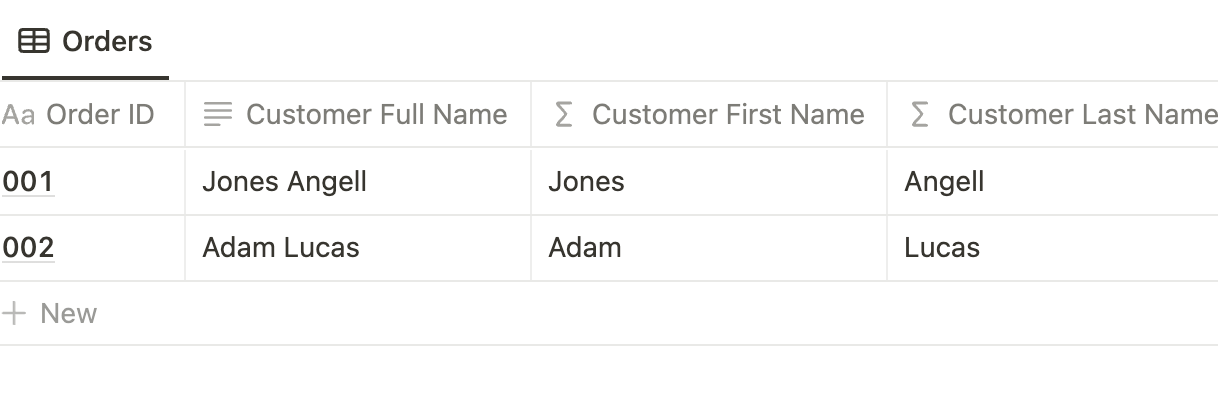Split the customer’s Full Name into First Name and Last Name properties.

### Formatting

Notion offers limited options for formatting numbers and dates. Rollup properties are especially constrained. With Formulas, you can control decimals and specify virtually any date or time format.

### Concatenation

Formulas can merge the values of other properties.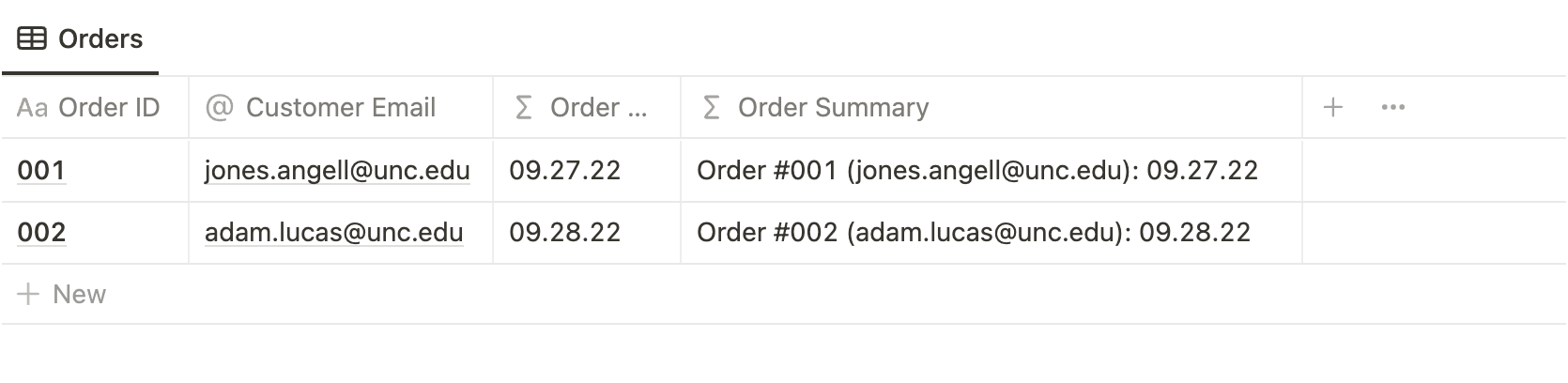Generate an Order Summary from the Order ID, Customer Email and reformatted Order Date.

## The Formula Window

Editing a Formula prompts the Formula Window. It contains three primary sections:

1. Compose the formula at the top.
2. The list at the left provides quick access to “ingredients” you may use in your formula, including functions and properties of your database.
3. With an element in that list highlighted, the bottom-right section offers an explanation.

## Ingredients of a Formula

You’ll compose formulas with two types of “ingredients”: input values and actions to perform on those values.

### Input Values

#### Data Types

Before diving into input values, let’s take a broader look at data types in Notion databases. Every value in a database, from simple `Title` and `Text` properties to specialized `Person` and `Multi-Select` properties, is one of four data types:

1. Number
2. String (text)
3. Date
4. Boolean (`true` or `false`)

Each of a formula’s input values is one of those four data types. So is the value returned by the formula.

It’s essential to remain mindful of data types because most actions must be performed on specific data types.

• You can’t divide a number by a text string; dividing requires two numbers.
• You can’t find the time between two numbers; they must be dates.
• When you use incompatible data types, you receive a `Type mismatch` error.

#### Literal Values

• Values you type directly into the formula, rather than a dynamic reference to another property.
• As the formula executes for each item in the database, it uses the same literal values.
• Text strings must be surrounded by double quotes: `"dog"`
• Dates cannot be supplied as literal values.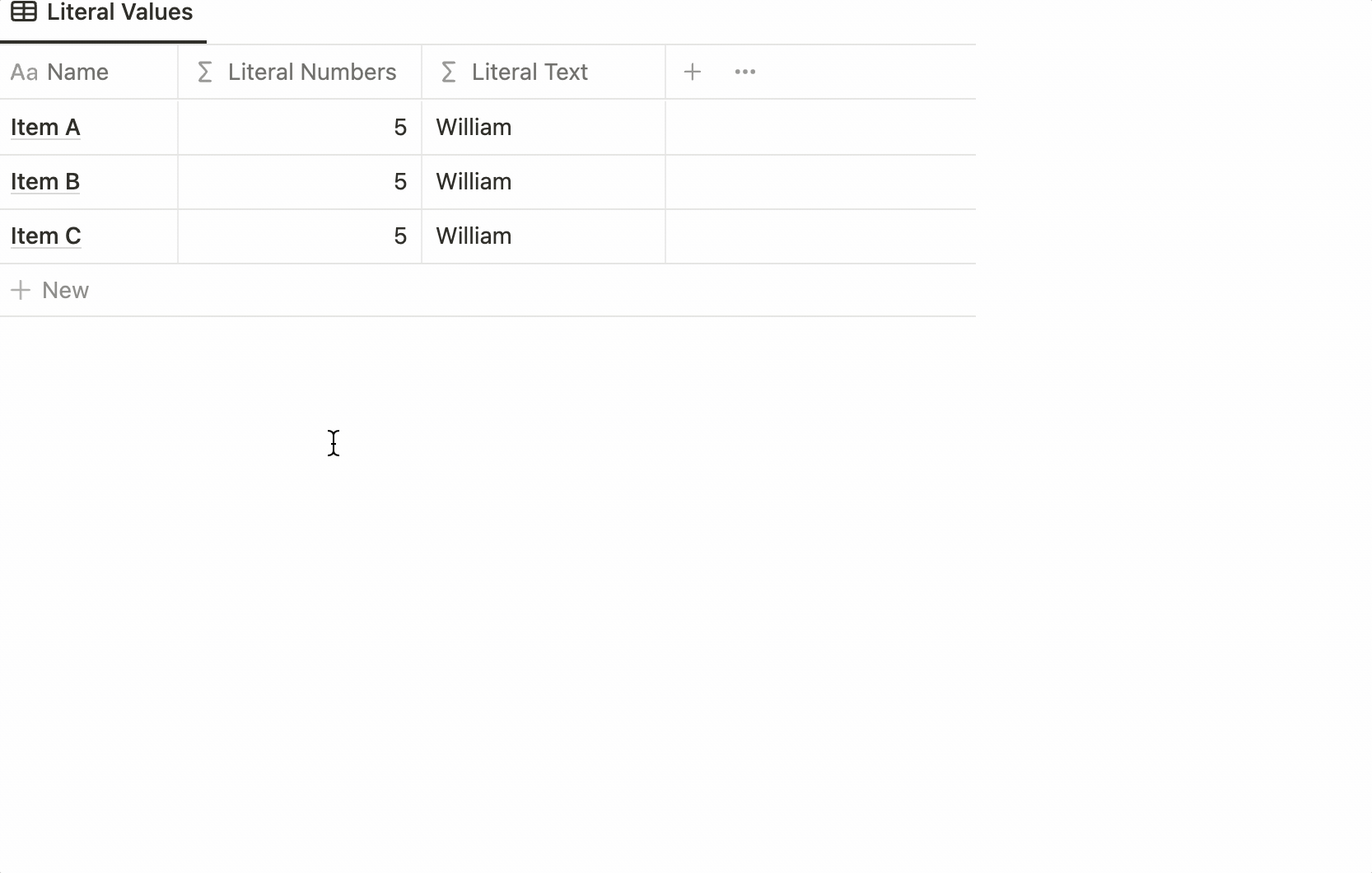Because the Formula properties use only literal inputs, the returned values are the same for all items of the database.

#### Constants

Notion offers a couple of keywords for mathematical constants, including `pi` and `e` (the base of the natural logarithm).

#### Property References

• Formulas typically reference other properties for their inputs.
• Unlike literal values and constants, which are the same for each item of the database, property references use the value of the referenced property for each item.
• Reference a property with the keyword `prop` followed by the property name quoted within parentheses: `prop("Property Name")`
• As a shortcut, you can choose properties from the left menu of the Formula Window.

### Actions

You’ll use two methods to define the actions to perform on your input values: operators and functions.

#### Operators

• Operators are one or two characters that define an action when placed between input values.
• They fall into three categories: arithmetic, comparison and concatenation.
• Notion A-to-Z includes a comprehensive index of operators by category.
##### Arithmetic Operators
• Arithmetic operators perform calculations predominately on numbers.
• You’ll find most of them familiar, such as `+` for adding and `/` for dividing.
##### Comparison Operators
• Comparison operators perform a test on one input against another.
• The returned value is either `true` or `false`, which Notion represents as a checked or unchecked `Checkbox`.
• Typically, the compared values must be of the same data type (number, string, date or boolean).
• You’re familiar with the operators such as `>` and `<=`. Use `==` to test equality or `!=` for inequality.
##### Concatenation Operator
• When placed between numbers, the `+` operator adds them. However, when placed between text strings, it concatenates, or merges, them.
• Remember: When entering text strings as literal values, surround them with double quotes: `"William Nutt"`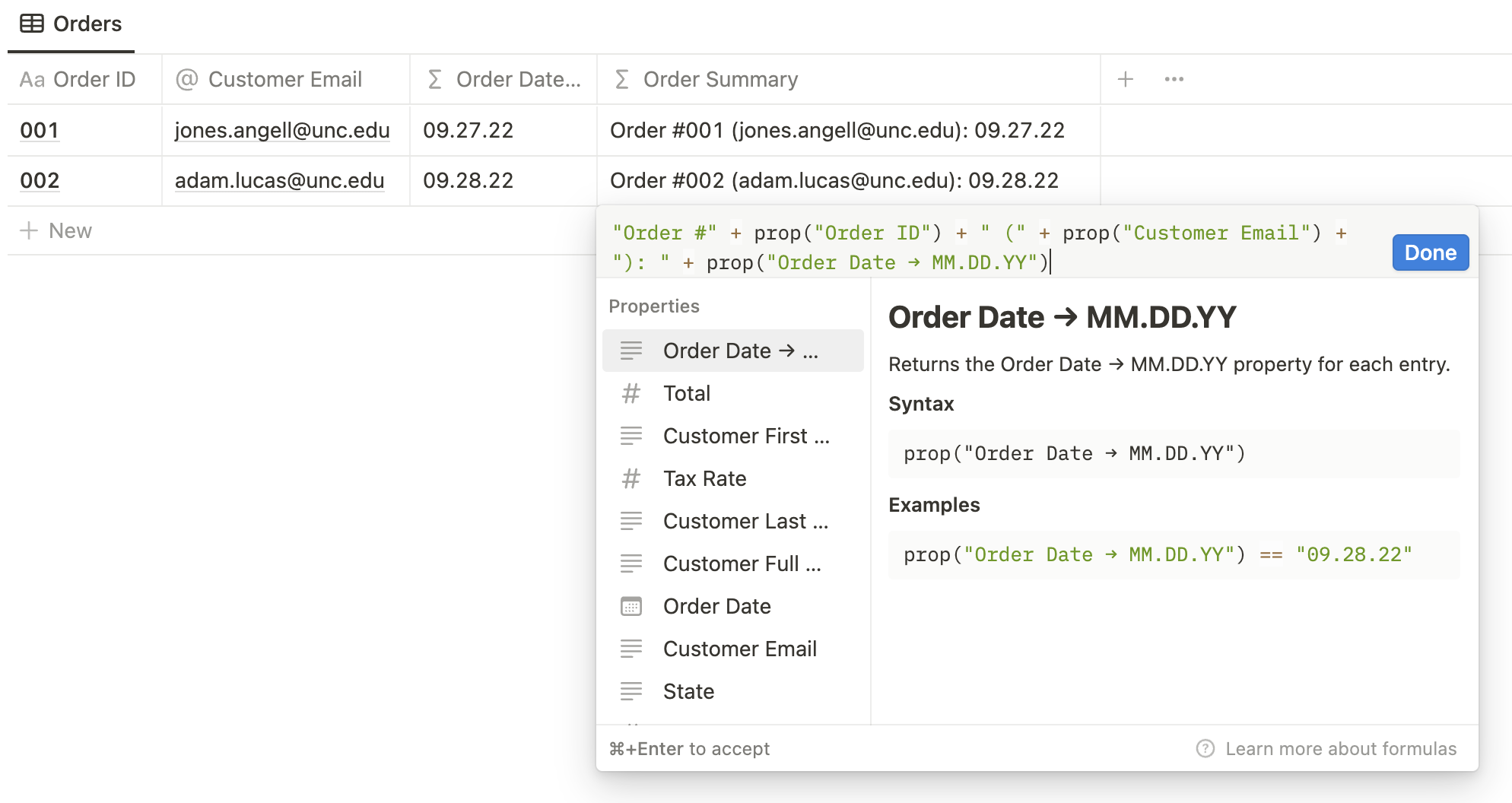Order Summary concatenates Order ID, Customer Email and Order Date → MM.DD.YY.

#### Functions

• Functions are like packaged actions to perform on inputs.
• Some simply replace operators, such as adding, multiplying and comparing.
• Other functions offer more advanced operations, such as converting data types, performing complex calculations, and defining conditional rules.
• The Formula Window lists all functions in the left menu. Hover over one to reveal helpful information to the right.
• You can click a function to add it to your formula, but for the sake of learning, I recommend writing them from scratch.
• My Notion Formula Cheat Sheet is more helpful. It’s among my most popular resources. I’m told the Notion team often references it internally.
##### Anatomy of a Function
• Each function begins with a keyword, which typically indicates its operation, such as `add`.
• That keyword is followed by parentheses containing your input values. These inputs are known as the function’s “arguments.”
• Each function has unique requirements for its arguments, which often include quantity and data type, such as numbers, dates or text. You’ll find those requirements when hovering over the function in the Formulas Window.
• For functions that accept more than one argument, you separate them with a comma.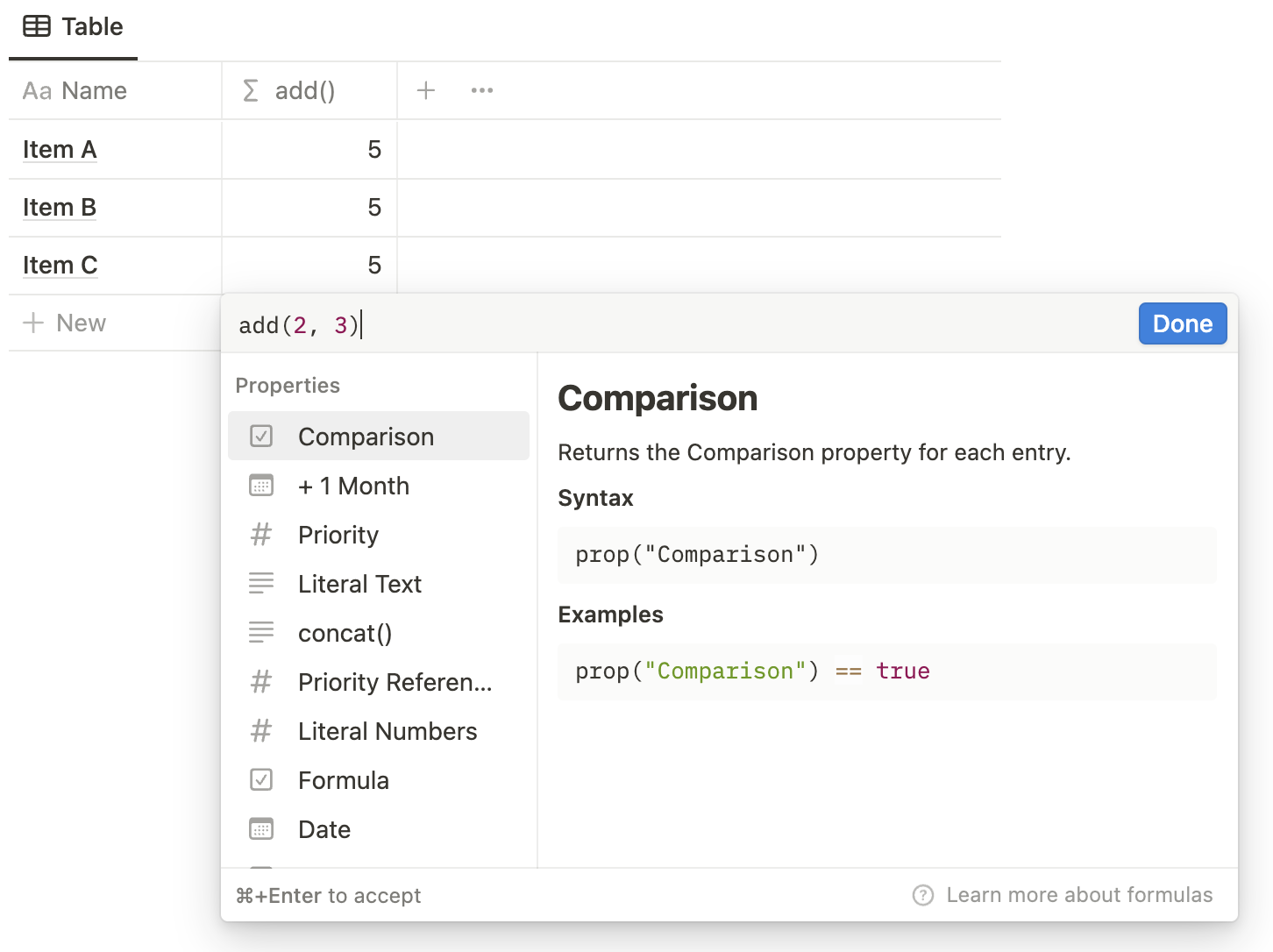add is the keyword. It accepts two arguments, which must be two numbers or text strings. 2 and 3 are supplied as those arguments.
##### Types of Functions

I group functions into four categories based on the data types they accept or return.

In our next training, we’ll explore common functions within each category. Here’s an introduction:

###### Numeric Functions
• Numeric functions work with `numbers` and mostly perform calculations.
• Each arithmetic operator has a corresponding function, such as `add()`.
• `round()`, `max()` and `min()` are other common numeric functions.
###### Text Functions
• Text functions generally manipulate text strings.
• The concatenation operator has a corresponding function, `concat()`, which merges the strings supplied as its arguments.
###### Date & Time Functions
• Date and time functions accept or return values of the `date` type.
• For use in these functions, a `date` value can originate in three ways:
1. A `Date` property
2. The `now()` function
3. The `fromTimestamp()` function
• `dateBetween()` and `dateAdd()` are common date functions.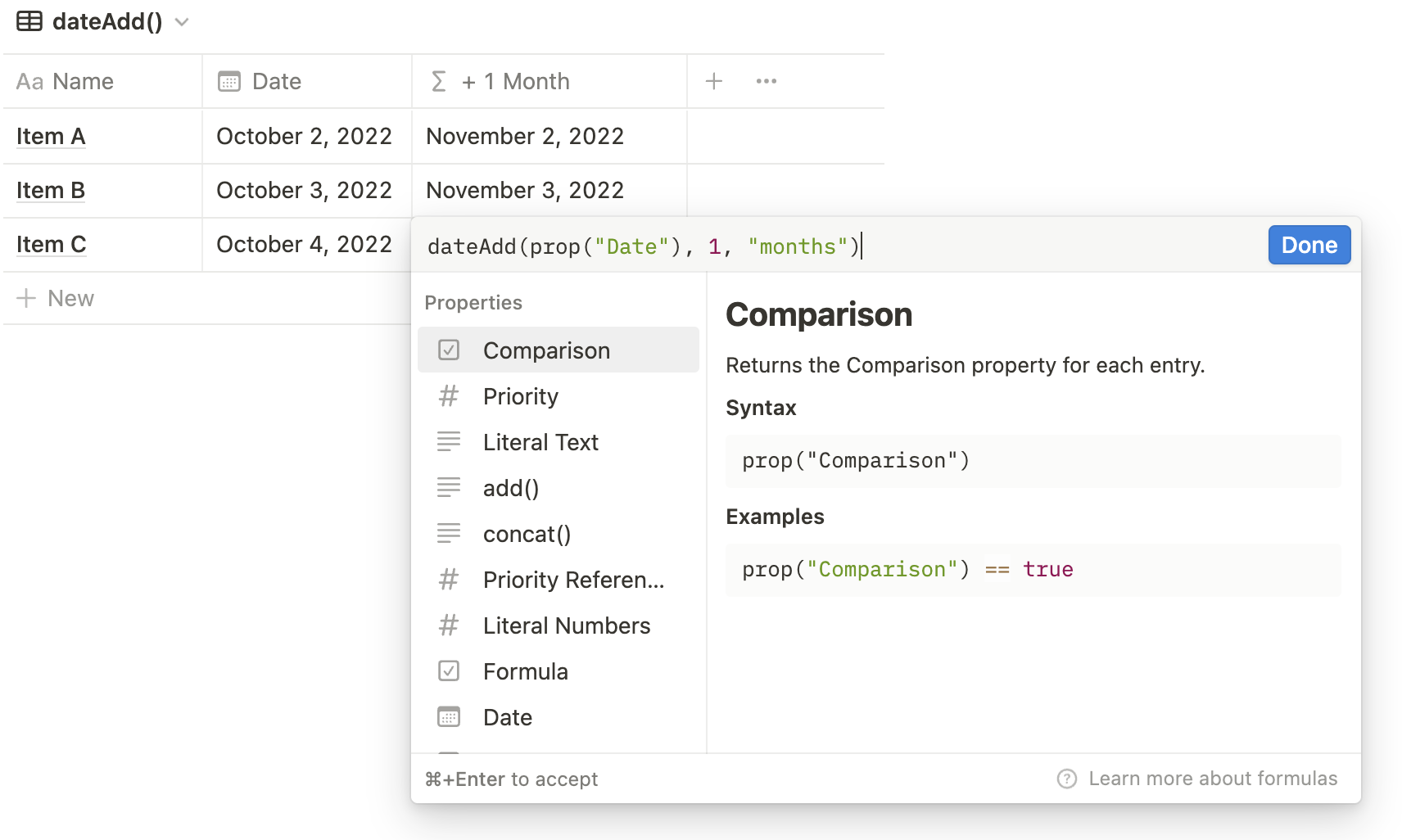The dateAdd() function accepts three arguments: (1) the initial date (the Date property above); (2) the quantity to add (1 above); (3) the unit, supplied as a lowercase, plural text string (”months”).
###### Logic Functions
• Logic functions make comparisons and define conditions.
• For every comparison operator, Notion offers a corresponding function.
• Those function fall within a dedicated section of the Formula Window.
• The most commonly used logic function, and one of the most commonly used functions overall, is `if()`, which we’ll save for the next workshop.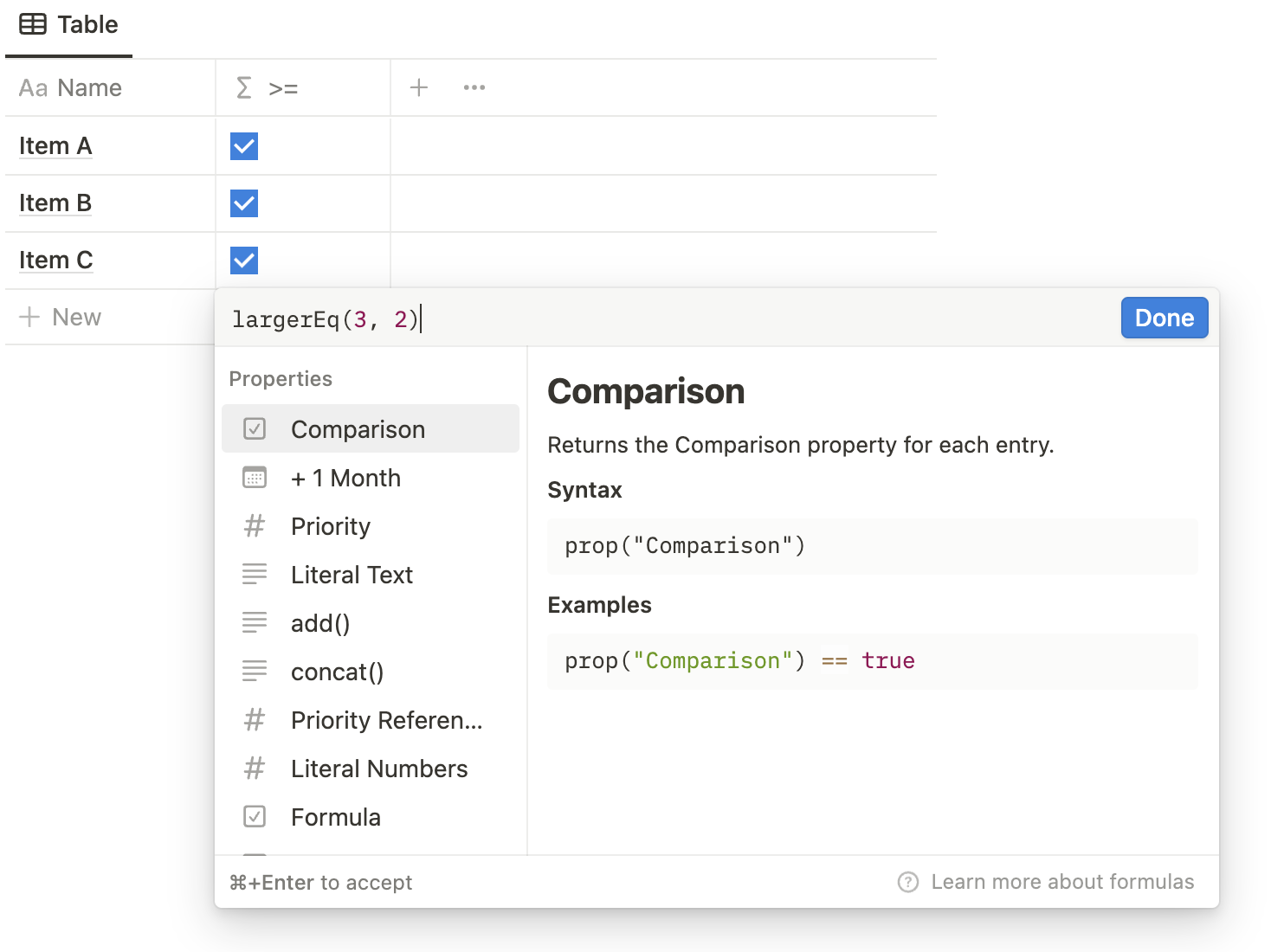The largerEq() function tests whether the first argument (6 below) is greater than or equal to the second argument (5 below).

### Practical Exercise

For this lesson’s practical exercise, the stakeholders created a database of expenses, with properties that employ the lesson’s concepts. The full instructions and final outcome are available to members of Notion A-to-Z.

All-in on
the all-in-one
productivity app.